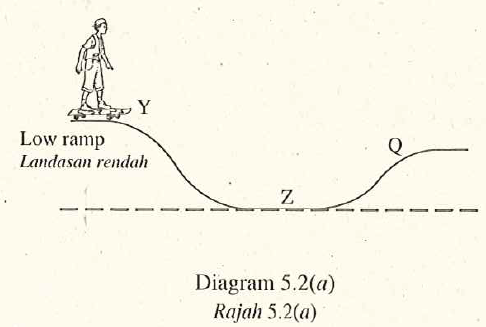# Structure Questions – Understanding Energy

Diagram 5.1 (a) shows a boy skating down a ramp from position $$X$$. Diagram 5.1 $$(b)$$ shows the velocity-time graph of the boy from $$X$$ to $$Z$$.Diagram $$5.2(a)$$ shows the same boy skating down from position $$Y$$ by using another ramp. Diagram $$5.2(b)$$ shows the velocity-time graph of the boy from $$Y$$ to $$Z$$.Both ramps are of the same slope and surface.

1. Name the physical quantity represented by the gradient of the velocity-time graph. [1 mark]
Acceleration
2. Observe Diagram 5.1 (a) and Diagram $$5.2(a)$$.
1. Compare the gravitational potential energy of the boy at $$X$$ and $$Y$$. [1 mark]
The gravitational potential energy in Diagram $$5.1$$ is higher than that of in Diagram 5.2.
2. Compare the velocity of the boy at $$Z$$ in these two situations. [1 mark]
The velocity of the boy at $$Z$$ in Diagram $$5.1$$ is higher than his velocity in Diagram 5.2.
3. Compare the kinetic energy of the boy at $$Z$$ in these two situations. [1 mark]
The kinetic energy of the boy at $$Z$$ is higher than his kinetic energy in Diagram $$5.2$$.
1. Based on the answer in $$5 (b)$$,
1. state the relationship between the gravitational potential energy and kinetic energy of the boy. [1 mark]
When gravitational potential energy increases, the kinetic energy increases.
2. state the physic concept involved. [1 mark]
Principle of conseryationi of energy
2. Based on Diagram $$5.1(a)$$,
1. what happens to the velocity of the boy when he skates from $$Z$$ to $$Q$$ ? [1 mark]
Decreases
2. Give one reason for the answer in $$5 (d)( i )$$. [1 mark]
The kinetic energy of the boy changes to potential energy.